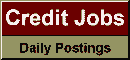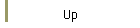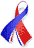DefaultRisk.com the web's biggest credit risk modeling resource.Export citation to:- HTML - Text (plain)- BibTeX - RIS - ReDIF

The Stochastic Intensity SSRD Model Implied Volatility Patterns for Credit Default Swap Options and the Impact of Correlation

by Damiano Brigo of Banca IMI, and
Laurent Cousot of Courant Institute

September 12, 2004

Abstract: In this paper we investigate implied volatility patterns in the Shifted Square Root Diffusion (SSRD) model as functions of the model parameters. We begin by recalling the Credit Default Swap (CDS) options market model that is consistent with a market Black-like formula, thus introducing a notion of implied volatility for CDS options. We examine implied volatilies coming from SSRD prices and characterize the qualitative behavior of implied volatilities as functions of the SSRD model parameters. We introduce an analytical approximation for the SSRD implied volatility that follows the same patterns in the model parameters and that can be used to have a first rough estimate of the implied volatility following a calibration. We compute numerically the CDS-rate volatility smile for the adopted SSRD model. We find a decreasing pattern of SSRD implied volatilities in the interest-rate/intensity correlation. We check whether it is possible to assume zero correlation after the option maturity in computing the option price and provide an upper bound for the Monte Carlo standard error in cases where this is not possible.

Keywords: Stochastic intensity model, credit default swap, calibration, option pricing, credit spread volatility, Monte Carlo simulation.

Published in: International Journal of Theoretical and Applied Finance, Vol. 9, No. 3, (May 2006), pp. 315-339.

Previously titled: A Comparison Between the Stochastic Intensity SSRD Model and the Market Model for CDS Options Pricing

Books Referenced in this paper:  (what is this?)